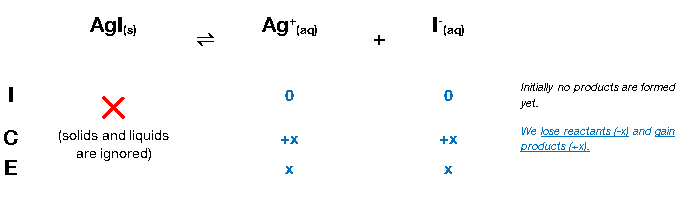# Problem: Calculate the molar solubility of AgI in pure water. K sp for AgI is 1.5 X 10 -16.

###### FREE Expert Solution

We can construct an ICE table for the dissociation of AgI

Remember that solids are ignored in the ICE table and Ksp expression.The Ksp expression for AgI is:

$\overline{){{\mathbf{K}}}_{{\mathbf{sp}}}{\mathbf{=}}\frac{\mathbf{products}}{\overline{)\mathbf{reactants}}}{\mathbf{=}}\mathbf{\left[}{\mathbf{Ag}}^{\mathbf{+}}\mathbf{\right]}\mathbf{\left[}{\mathbf{I}}^{\mathbf{-}}\mathbf{\right]}}$

Note that each concentration is raised by the stoichiometric coefficient: [Ag+] and [I] are raised to 1

We can now plug in the equilibrium concentrations and solve for x, which is the molar solubility of AgI

100% (420 ratings)###### Problem Details

Calculate the molar solubility of AgI in pure water. K sp for AgI is 1.5 X 10 -16.